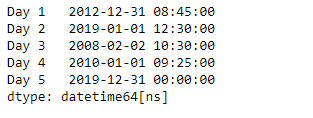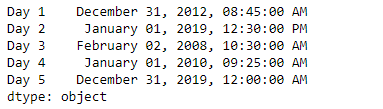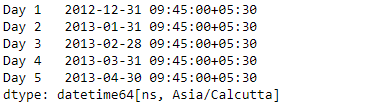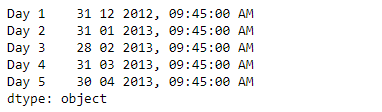Skip to content
Related Articles
Python | Pandas Series.dt.strftime
• Difficulty Level : Easy
• Last Updated : 20 Mar, 2019

`Series.dt` can be used to access the values of the series as datetimelike and return several properties. Pandas` Series.dt.strftime()` function is used to convert to Index using specified date_format. The function return an Index of formatted strings specified by date_format, which supports the same string format as the python standard library.

Syntax: Series.dt.strftime(*args, **kwargs)

Parameter :
date_format : Date format string (e.g. “%Y-%m-%d”)

Returns : Index of formatted strings

Example #1: Use `Series.dt.strftime()` function to convert the dates in the given series object to the specified date format.

 `# importing pandas as pd``import` `pandas as pd`` ` `# Creating the Series``sr ``=` `pd.Series([``'2012-12-31 08:45'``, ``'2019-1-1 12:30'``, ``'2008-02-2 10:30'``,``               ``'2010-1-1 09:25'``, ``'2019-12-31 00:00'``])`` ` `# Creating the index``idx ``=` `[``'Day 1'``, ``'Day 2'``, ``'Day 3'``, ``'Day 4'``, ``'Day 5'``]`` ` `# set the index``sr.index ``=` `idx`` ` `# Convert the underlying data to datetime ``sr ``=` `pd.to_datetime(sr)`` ` `# Print the series``print``(sr)`

Output :Now we will use `Series.dt.strftime()` function to convert the dates in the given series object to the specified format.

 `# convert to the given date format``result ``=` `sr.dt.strftime(``'% B % d, % Y, % r'``)`` ` `# print the result``print``(result)`

Output :As we can see in the output, the `Series.dt.strftime()` function has successfully converted the dates in the given series object to the specified format.

Example #2 : Use `Series.dt.strftime()` function to convert the dates in the given series object to the specified date format.

 `# importing pandas as pd``import` `pandas as pd`` ` `# Creating the Series``sr ``=` `pd.Series(pd.date_range(``'2012-12-31 09:45'``, periods ``=` `5``, freq ``=` `'M'``,``                            ``tz ``=` `'Asia / Calcutta'``))`` ` `# Creating the index``idx ``=` `[``'Day 1'``, ``'Day 2'``, ``'Day 3'``, ``'Day 4'``, ``'Day 5'``]`` ` `# set the index``sr.index ``=` `idx`` ` `# Print the series``print``(sr)`

Output :Now we will use `Series.dt.strftime()` function to convert the dates in the given series object to the specified format.

 `# convert to the given date format``result ``=` `sr.dt.strftime(``'% d % m % Y, % r'``)`` ` `# print the result``print``(result)`

Output :As we can see in the output, the `Series.dt.strftime()` function has successfully converted the dates in the given series object to the specified format.

Attention geek! Strengthen your foundations with the Python Programming Foundation Course and learn the basics.

To begin with, your interview preparations Enhance your Data Structures concepts with the Python DS Course. And to begin with your Machine Learning Journey, join the Machine Learning – Basic Level Course

My Personal Notes arrow_drop_up